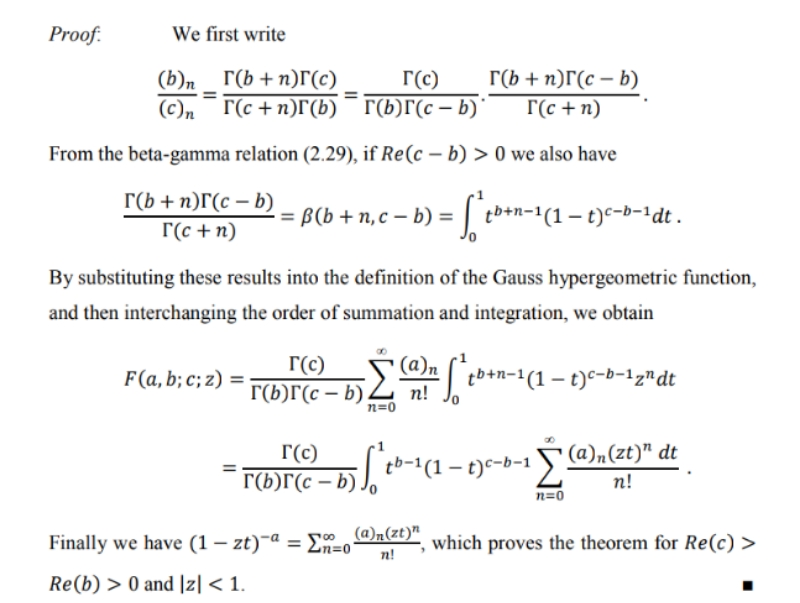# $π$

$\sum_{n=0}^∞ \frac{((\frac{1}{2})_n)^2 }{(2)_n .n!} = _2F_1 (\frac{1}{2},\frac{1}{2};2;1)= \frac{1}{\Gamma^2{(\frac{3}{2})}}= \frac{4}{π}$ $\sum_{n=0}^∞ \frac{(\frac{2}{3})_n (\frac{1}{3})_n }{(2)_n .n!}= _2F_1 (\frac{2}{3},\frac{1}{3};2;1) =\frac{9\sqrt{3}}{4π}$ $\sum_{n=0}^∞ \frac{(\frac{3}{4})_n (\frac{1}{4})_n}{(2)_n .n!}= _2F_1(\frac{3}{4} , \frac{1}{4} ;2;1)= \frac{8\sqrt{2}}{3π}$ $\sum_{n=0}^∞ \frac{(\frac{4}{5})_n (\frac{1}{5})_n }{(2)_n .n!} = _2F_1(\frac{1}{5},\frac{4}{5} ;2;1) = \frac{25\sin{(\frac{π}{5})}}{4π}$

$\textrm{And many more...}$

In all cases I have used $_2F_1 (a,b;c;1)= \frac{\Gamma{(c)}\Gamma{(c-a-b)}}{\Gamma{(c-a)}\Gamma{(c-b)}}$

I have noticed that , we can easily found out an Infinite sum generator for $π$

When $\color{#20A900}_2F_1 (1-\frac{1}{a} , \frac{1}{a} ;2;1) = \frac{a^2 \sin(\frac{π}{a})}{(a-1)π}$ When $a=97$ then it is $\large\frac{9409}{96π} \sin(\frac{π}{97})$

Those Expressions can be transformed into infinite sums . For example

$_2F_1(\frac{1}{2},\frac{1}{2};2;1)= 1+\frac{1}{2} (\frac{1}{2.1!})^2+\frac{1}{3} (\frac{1.3}{2^2.2!})^2 +\frac{1}{4}(\frac{1.3.5}{2^3.3!})^2 +\cdots$

Proof of Above IdentityTake $z=1$ , then it becomes $_2F_1 (a,b;c,1)= \frac{\Gamma{(c)}}{\Gamma{(b)}\Gamma{(c-b)} } \int_0^1 t^{b-1} (1-t)^{c-b-a-1}dt$ $= \frac{\Gamma{(c)}}{\Gamma{(b)}\Gamma{(c-b)}} \Beta{(b,c-b-a)} = \frac{\Gamma{(c)} \Gamma{(c-a-b)}}{\Gamma{(c-a)}\Gamma{(c-b)}}$Note by Dwaipayan Shikari
6 months, 2 weeks ago

This discussion board is a place to discuss our Daily Challenges and the math and science related to those challenges. Explanations are more than just a solution — they should explain the steps and thinking strategies that you used to obtain the solution. Comments should further the discussion of math and science.

When posting on Brilliant:

• Use the emojis to react to an explanation, whether you're congratulating a job well done , or just really confused .
• Ask specific questions about the challenge or the steps in somebody's explanation. Well-posed questions can add a lot to the discussion, but posting "I don't understand!" doesn't help anyone.
• Try to contribute something new to the discussion, whether it is an extension, generalization or other idea related to the challenge.

MarkdownAppears as
*italics* or _italics_ italics
**bold** or __bold__ bold
- bulleted- list
• bulleted
• list
1. numbered2. list
1. numbered
2. list
Note: you must add a full line of space before and after lists for them to show up correctly
paragraph 1paragraph 2

paragraph 1

paragraph 2

[example link](https://brilliant.org)example link
> This is a quote
This is a quote
    # I indented these lines
# 4 spaces, and now they show
# up as a code block.

print "hello world"
# I indented these lines
# 4 spaces, and now they show
# up as a code block.

print "hello world"
MathAppears as
Remember to wrap math in $$ ... $$ or $ ... $ to ensure proper formatting.
2 \times 3 $2 \times 3$
2^{34} $2^{34}$
a_{i-1} $a_{i-1}$
\frac{2}{3} $\frac{2}{3}$
\sqrt{2} $\sqrt{2}$
\sum_{i=1}^3 $\sum_{i=1}^3$
\sin \theta $\sin \theta$
\boxed{123} $\boxed{123}$

Sort by:

Very nice! Put in it Percy's Tau discussion!

- 6 months, 1 week ago

I will add more values later :)

- 6 months, 2 weeks ago